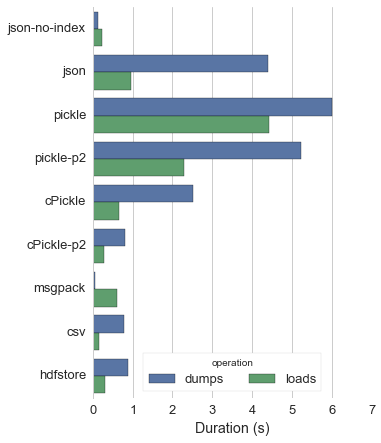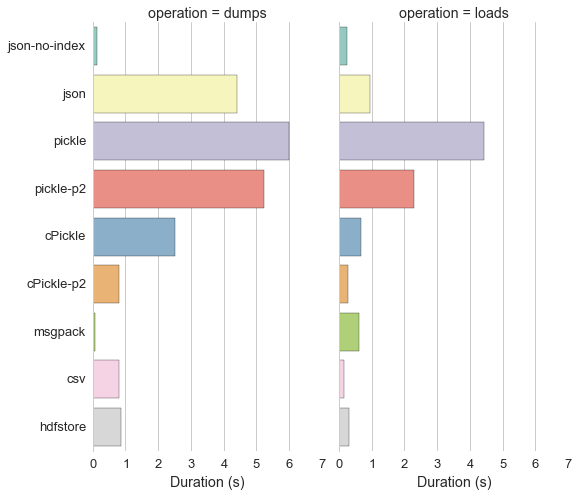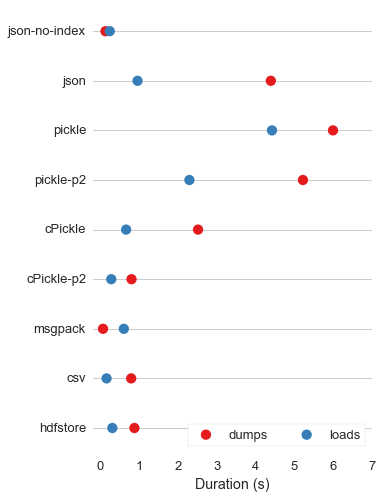In :
%matplotlib inline

In :
import seaborn as sns
import pandas as pd
import matplotlib.pyplot as plt


Note that this is using current seaborn master:

In :
print sns.__version__

0.6.dev


I think the "whitegrid" style looks best with barplots and other plots that have large patches of color.

In :
sns.set(style="whitegrid", font_scale=1.3)

In :
df = pd.read_csv(("https://gist.githubusercontent.com/mrocklin/56830db18bd71ba7d9e3/"

In :
df.index.name = "storage"
df = df.reset_index()

In :
df.head()

Out:
0 json-no-index 0.129610 0.239696
1 json 4.397093 0.957318
2 pickle 6.001169 4.426793
3 pickle-p2 5.225624 2.295486
4 cPickle 2.518837 0.662841

Melt the dataframe to long-form.

In :
df = pd.melt(df, "storage", value_name="duration", var_name="operation")

In :
df.head()

Out:
storage operation duration
0 json-no-index dumps 0.129610
1 json dumps 4.397093
2 pickle dumps 6.001169
3 pickle-p2 dumps 5.225624
4 cPickle dumps 2.518837

### Option 1: a horizontal nested bar plot¶

Drawing the bars horizontally works best when the category values are long strings, as they don't overlap or have to get awkwardly rotated.

Stacked bar plots can be hard to read and don't work all that well except in certain circumstances, so we'll draw separate plots for each operation.

Using nested plots emphasizes the comparison between the two operations for each storage option, but makes it slightly harder to compare across storage options for a given operation (though still easier than with stacked bar plots):

In :
f, ax = plt.subplots(figsize=(5, 7))

# Draw the plot
sns.barplot("duration", "storage", "operation", data=df)

# The default legend doesn't look great here
# let's move it and add a frame and title
ax.legend(loc="lower center", ncol=2, frameon=True, title="operation")

# Seaborn automatically labels the axes with the variable names
# which can be useful, but for production plots it' usually good
# to tweak them. Let's add units to the x axis label and remove
# the y axis label, which is not overly necessary and takes up space.
ax.set(xlabel="Duration (s)", ylabel="")

# Removing the axes spines gives a cleaner look
sns.despine(bottom=True)### Option 2: faceted bar plot¶

The other option would be to make two plots, one for each operation. This will make it easier to compare across storage options within each operation, but it will be somewhat more difficult to compare across operations within each storage option.

In :
g = sns.factorplot("duration", "storage", col="operation", data=df,
kind="bar", size=7, aspect=.6, palette="Set3")
g.set_axis_labels("Duration (s)", "")
g.despine(bottom=True)

Out:
<seaborn.axisgrid.FacetGrid at 0x1091c0510>### Option 3: dot plot¶

Another option would be a dot plot, which makes it easy to compare both across operations and across storage.

In :
f, ax = plt.subplots(figsize=(5, 8))

# Draw the plot
sns.pointplot("duration", "storage", "operation", data=df,
join=False, palette="Set1")

# The default legend doesn't look great here
# let's move it and add a frame and title
ax.legend(loc="lower right", ncol=2, frameon=True)

# Seaborn automatically labels the axes with the variable names
# which can be useful, but for production plots it' usually good
# to tweak them. Let's add units to the x axis label and remove
# the y axis label, which is not overly necessary and takes up space.
ax.set(xlabel="Duration (s)", ylabel="",
xlim=(-.2, None))

# A dot plot looks better with a grid on the categorical axis
# to help connect each point to its label. Removing the grid on the
# numerical axis makes the plot cleaner, though it is a bit hard to
# read off exact values for each point.
ax.xaxis.grid(False)
ax.yaxis.grid(True)

# Removing the axes spines gives a cleaner look
sns.despine(left=True, bottom=True)In [ ]: